Next: Worked example 3.1: Broken Up: Motion in 3 dimensions Previous: Projectile motion

## Relative velocity

Suppose that, on a windy day, an airplane moves with constant velocity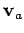with respect to the air, and that the air moves with constant velocity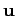with respect to the ground. What is the vector velocity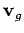of the plane with respect to the ground? In principle, the answer to this question is very simple: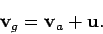(81)

In other words, the velocity of the plane with respect to the ground is the vector sum of the plane's velocity relative to the air and the air's velocity relative to the ground. See Fig. 18. Note that, in general,is parallel to neithernor. Let us now consider how we might implement Eq. (81) in practice.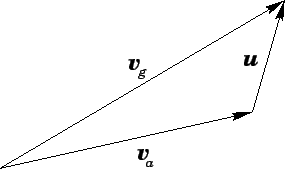As always, our first task is to set up a suitable Cartesian coordinate system. A convenient system for dealing with 2-dimensional motion parallel to the Earth's surface is illustrated in Fig. 19. The-axis points northward, whereas the-axis points eastward. In this coordinate system, it is conventional to specify a vector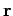in term of its magnitude,, and its compass bearing,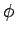. As illustrated in Fig. 20, a compass bearing is the angle subtended between the direction of a vector and the direction to the North pole: i.e., the-direction. By convention, compass bearings run fromto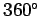. Furthermore, the compass bearings of North, East, South, and West are,,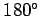, and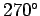, respectively.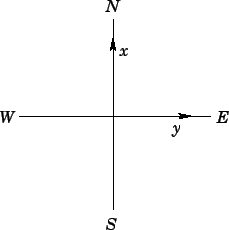According to Fig. 20, the components of a general vector, whose magnitude isand whose compass bearing is, are simply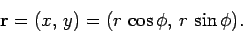(82)

Note that we have suppressed the-component of(which is zero), for ease of notation. Although, strictly speaking, Fig. 20 only justifies the above expression forin the rangeto, it turns out that this expression is generally valid: i.e., it is valid forin the full rangeto.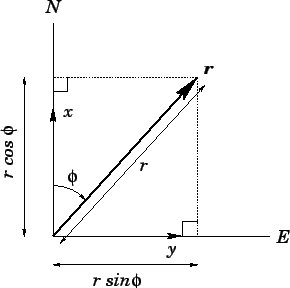As an illustration, suppose that the plane's velocity relative to the air is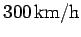, at a compass bearing of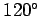, and the air's velocity relative to the ground is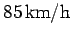, at a compass bearing of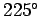. It follows that the components ofand(measured in units of km/h) are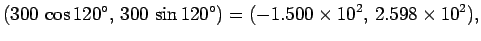(83)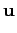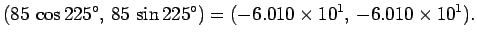(84)

According to Eq. (81), the components of the plane's velocityrelative to the ground are simply the algebraic sums of the corresponding components ofand. Hence,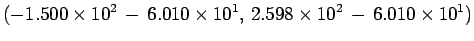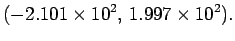(85)

Our final task is to reconstruct the magnitude and compass bearing of vector, given its components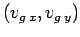. The magnitude offollows from Pythagoras' theorem [see Eq. (35)]: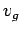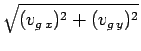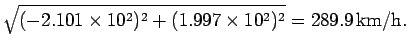(86)

In principle, the compass bearing ofis given by the following formula:(87)

This follows because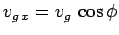and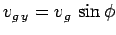[see Eq. (82)]. Unfortunately, the above expression becomes a little difficult to interpret if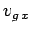is negative. An unambiguous pair of expressions foris given below: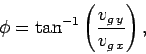(88)

if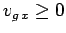; or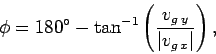(89)

if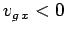. These expressions can be derived from simple trigonometry. For the case in hand, Eq. (89) is the relevant expression, hence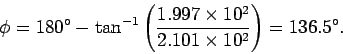(90)

Thus, the plane's velocity relative to the ground is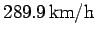at a compass bearing of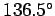.Next: Worked example 3.1: Broken Up: Motion in 3 dimensions Previous: Projectile motion
Richard Fitzpatrick 2006-02-02